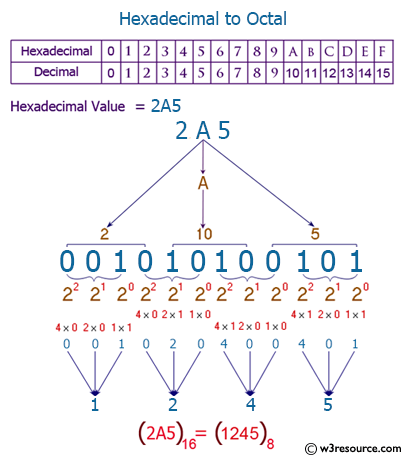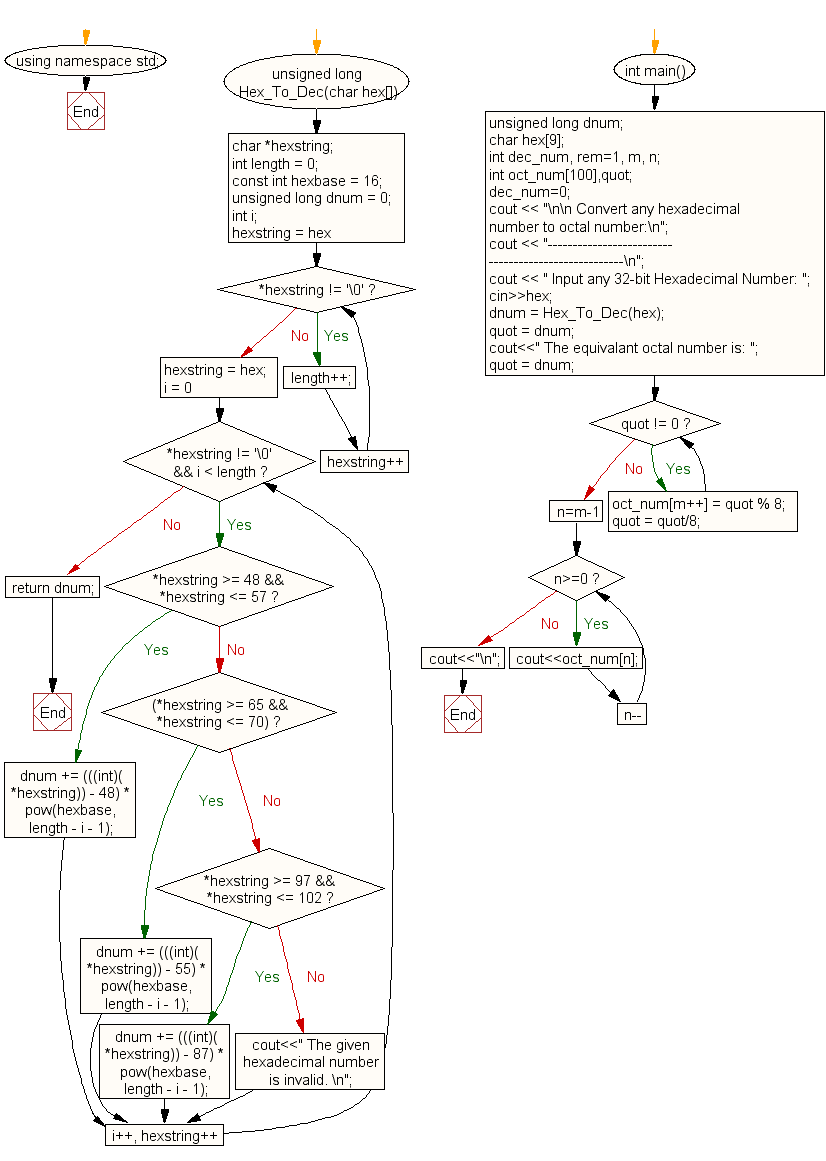﻿ C++ Exercises: Convert a hexadecimal number to octal number - w3resource# C++ Exercises: Convert a hexadecimal number to octal number

## C++ For Loop: Exercise-81 with Solution

Write a program in C++ to convert a hexadecimal number to octal number.

Pictorial Presentation:Sample Solution:-

C++ Code :

``````#include<iostream>
#include<stdlib.h>
#include<math.h>
using namespace std;

unsigned long Hex_To_Dec(char hex[])
{
char *hexstring;
int length = 0;
const int hexbase = 16;
unsigned long dnum = 0;
int i;
for (hexstring = hex; *hexstring != '\0'; hexstring++)
{
length++;
}
hexstring = hex;
for (i = 0; *hexstring != '\0' && i < length; i++, hexstring++)
{
if (*hexstring >= 48 && *hexstring <= 57)
{
dnum += (((int)(*hexstring)) - 48) * pow(hexbase, length - i - 1);
}
else if ((*hexstring >= 65 && *hexstring <= 70))
{
dnum += (((int)(*hexstring)) - 55) * pow(hexbase, length - i - 1);
}
else if (*hexstring >= 97 && *hexstring <= 102)
{
dnum += (((int)(*hexstring)) - 87) * pow(hexbase, length - i - 1);
}
else {
cout<<" The given hexadecimal number is invalid. \n";
}
}
return dnum;
}

int  main()
{
unsigned long dnum;
char hex;
int dec_num, rem=1, m, n;
int oct_num,quot;
dec_num=0;
cout << "\n\n Convert any hexadecimal number to octal number:\n";
cout << "----------------------------------------------------\n";
cout << " Input any 32-bit Hexadecimal Number: ";
cin>>hex;
dnum = Hex_To_Dec(hex);
quot = dnum;
cout<<" The equivalent octal number is: ";
quot = dnum;
while(quot != 0)
{
oct_num[m++] = quot % 8;
quot = quot/8;
}
for(n=m-1; n>=0; n--)
{
cout<<oct_num[n];
}
cout<<"\n";

}
``````

Sample Output:

``` Convert any hexadecimal number to octal number:
----------------------------------------------------
Input any 32-bit Hexadecimal Number: 5f
The equivalant octal number is: 137
```

Flowchart:C++ Code Editor:

Contribute your code and comments through Disqus.

What is the difficulty level of this exercise?

﻿